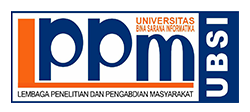### SIMULASI KENDALI MUTU DOSIS OBAT BERBASIS BOBOT DENGAN MENGGUNAKAN LOGIKA FUZZY

Panca Hariwan

#### Abstract

This fuzzy logic system has 3 input parameters such as thickness, hardness, diameter and has a single output that is hard tablets. From the input and output has three linguistic labels for each membership function, and has if-then rules used in this fuzzy system. Fuzzy algorithm can be used as an algorithm to determine the level of thickness, hardness, and the diameter of a tablet. In this study Mamdani model is used to control the tablet weight of each of these three parameters change tablets. Input membership function of the parameter determines the level of the tablet weight deviation from the nominal value of tablets. Results weight tablets using fuzzy logic with rule-based approach and common sense have a stable average rate of 99,953%. In the production process that uses pencetakkan tablets fuzzy logic Mamdani model occurs only deviation: 606 mg (max), 600 mg (min), 600 mg (standard), which gained an average of 600,283 mg + 0047%.

Keywords: fuzzy, production, tablets, MATLAB

#### Full Text:

PDF

DOI: https://doi.org/10.31294/p.v12i1.3471

ISSN2579-3500